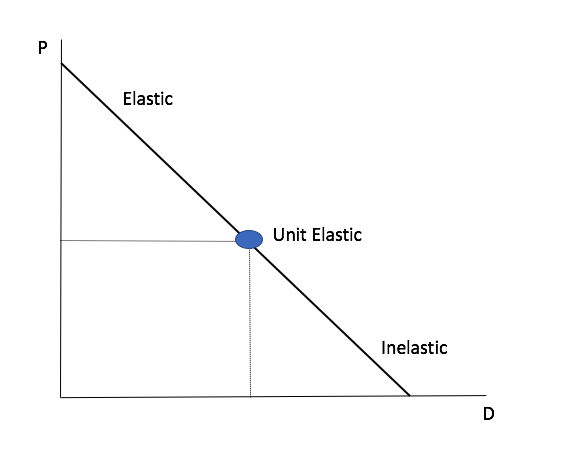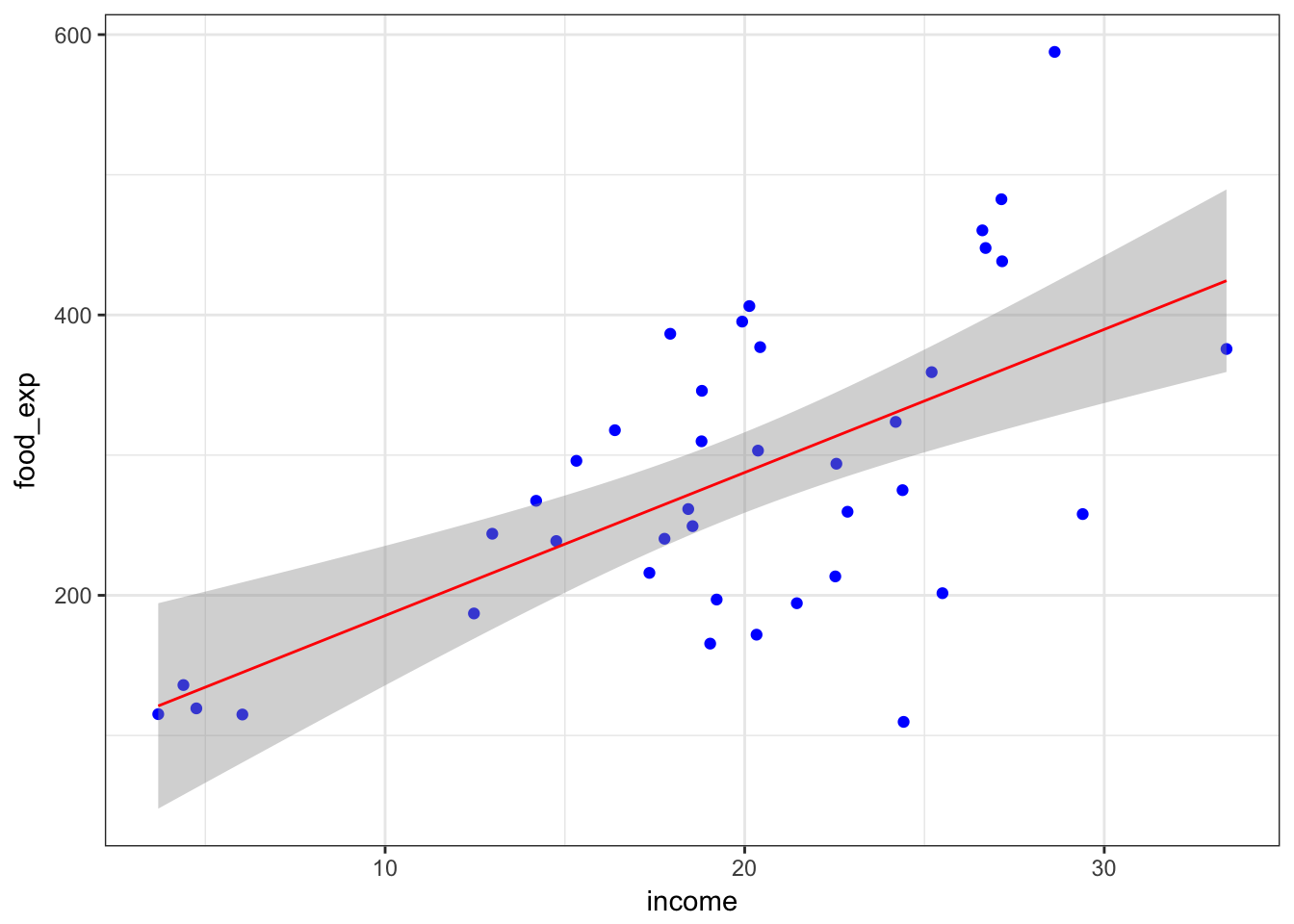## Price Elasticity of Defections

The price elasticity of defections (PED) is a measure of the relationship between a change in quantity of defections as a result of a change in service price. A small change in service price that results in a large change in quantity of defections is said to be elastic. Conversely, a large change in price accompanied by a small change in quantity of defections is said to be inelastic. If a change in price is accompanied by an equal change in quantity of defections, then PED is said to be unit elastic. That is,

• If $$\text{PED} < \lvert 1 \rvert \to \text{inelastic}$$
• If $$\text{PED} > \lvert 1 \rvert \to \text{elastic}$$
• If $$\text{PED} = \lvert 1 \rvert \to \text{unit elastic}$$

If we let $$P$$ denote service price and let $$D$$ denote the quantity of defections, then the price elasticity of defections can be computed as follows

\begin{aligned} \epsilon_{d} & = \frac{\%\Delta D }{\%\Delta P} \\\\ & = \frac{\Delta D / D}{\Delta P / P} = \frac{\Delta D}{D} \frac{P}{\Delta P} \\\\ & = \frac{\Delta D}{\Delta P} \frac{P}{D} \end{aligned}Price Elasticity Curve for Defections

Figure 1 is an extremely helpful graphical representation of price elasticity in that it allows us to estimate the service price point at which customers will defect.

## Using OLS Linear Regression to Calculate PED

We can calculate the price elasticity of a good by creating a linear regression model. In addition to being able to determine the price elasticity of defections, we can also predict the quantity of defections for a given service price! A linear regression primer is beyond the scope of this document. However, in general, linear regression models represent the linear relationship between a dependent variable and one or more independent variables. Mathematically, the general form of a linear regression model with one independent variable can be expressed as

\begin{aligned} y = \beta_{0} + \beta_{1}x + e \end{aligned}

where $$y$$ is the dependent variable, $$x$$ is the independent variable, $$\beta_{0}$$ is the intercept, $$\beta_{1}$$ is the coefficient of $$x$$, and $$e$$ is an unobserved random error term. Our goal is to estimate the parameters $$\beta_{0}$$ and $$\beta_{1}$$. Thus form of the fitted model becomes

\begin{aligned} \hat{y}_{i} = b_{0} + b_{1}x_{i} \end{aligned}

### An Example Using Food Expenditure Data

Using a data set on food expenditures against income, we can estimate the price (income, in this case) elasticity of food expenditures. That is, how sensitive is the change in a household’s food expenditures based on a change in income.

library(foreign)

# examine our data
summary(food)
##     food_exp         income
##  Min.   :109.7   Min.   : 3.69
##  1st Qu.:200.4   1st Qu.:17.11
##  Median :264.5   Median :20.03
##  Mean   :283.6   Mean   :19.60
##  3rd Qu.:363.3   3rd Qu.:24.40
##  Max.   :587.7   Max.   :33.40

Creating a scatter plot is always a good step for exploratory data analysis. Here, food expenditure is our dependent variable and income is our independent variable. Let’s plot food expenditure against income along with a fitted regression line.

library(ggplot2)
theme_set(theme_bw())

ggplot(data = food, aes(y = food_exp, x = income))  + geom_point(col = 'blue') + geom_smooth(method = 'lm', col = 'red', size = 0.5) # fitted regression lineAs you can see from the scatter plot, there seems to be a positive linear relationship between food expenditure and income, as one would suspect. Now, let’s fit our model.

food.lm <- lm(food_exp ~ income, data = food)
summary(food.lm)
##
## Call:
## lm(formula = food_exp ~ income, data = food)
##
## Residuals:
##      Min       1Q   Median       3Q      Max
## -223.025  -50.816   -6.324   67.879  212.044
##
## Coefficients:
##             Estimate Std. Error t value Pr(>|t|)
## (Intercept)   83.416     43.410   1.922   0.0622 .
## income        10.210      2.093   4.877 1.95e-05 ***
## ---
## Signif. codes:  0 '***' 0.001 '**' 0.01 '*' 0.05 '.' 0.1 ' ' 1
##
## Residual standard error: 89.52 on 38 degrees of freedom
## Multiple R-squared:  0.385,  Adjusted R-squared:  0.3688
## F-statistic: 23.79 on 1 and 38 DF,  p-value: 1.946e-05

#### Interpreting the Results

Again, the purpose of this document is not to explain linear regression in depth. Rather, focus on the values for “Estimate.” Those values are our parameter estimates. That is, they are the values for $$b_{0}$$ (intercept) and $$b_{1}$$ (coefficient of $$x$$). Thus, the equation for our fitted model is

\begin{aligned} \widehat{\text{FOOD_EXP}} = 83.416 + 10.21 \times \text{INCOME} \end{aligned}

Interpreting the coefficient is very important! What the equation tells us that a one-unit increase in income results in a 10.21-dollar increase in food expenditures. Because the coefficient $$\beta_{1}$$ represents the change in the dependent variable $$y$$ with respect to $$x$$ (i.e. $$\Delta y / \Delta x$$), we can write the elasticity of food expenditures as

\begin{aligned} \epsilon = \frac{\Delta y / y}{\Delta x / x} = \frac{\Delta y}{\Delta x} \cdot \frac{x}{y} = \beta_{1} \cdot \frac{x}{y} \end{aligned}

To calculate elasticity, we need values for $$x$$ and $$y$$, or income and food expenditures in this case. We can use their mean values, which are $$19.6$$ and $$283.6$$, respectively. Thus, we have

\begin{aligned} \epsilon = 10.21 \times \frac{19.6}{283.6} = 0.71 \end{aligned}

In this example, because PED $$< \lvert 1 \rvert$$, the income elasticity of food expenditures is inelastic. That is, a one percent change in income results in a less than one percent change in food expenditures.